Home » Blog » 2nd Grade Skills » Geometry » Math Puzzles in the Classroom

# Math Puzzles in the Classroom

Written by: Angie Olson

Teachers are always looking for ways for their students to practice math skills.  Math puzzles are a great option because they are engaging, independent, self-correcting, and easy to prep.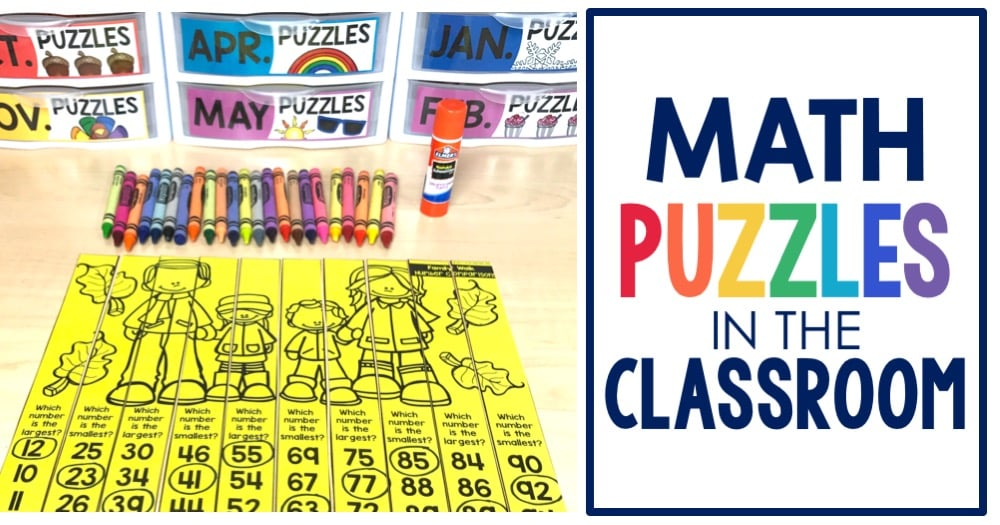## What are Math Puzzles

Math puzzles are a hands on option for students to practice their math skills.  Math puzzles are available in black and white, full color, editable, and student scrambled.

## When to use Math Puzzles

Math puzzles are great to use during math centers, as an early finisher, intervention activity, or even a math enrichment activity.  For more math centers ideas read this post.

## How to use Math Puzzles

Students solve the math problem on each puzzle piece.  The answer gets written in the circle provided on the puzzle piece.  Then the student arranges the answers in order from least to greatest.  Once finished, the math puzzle will display a picture.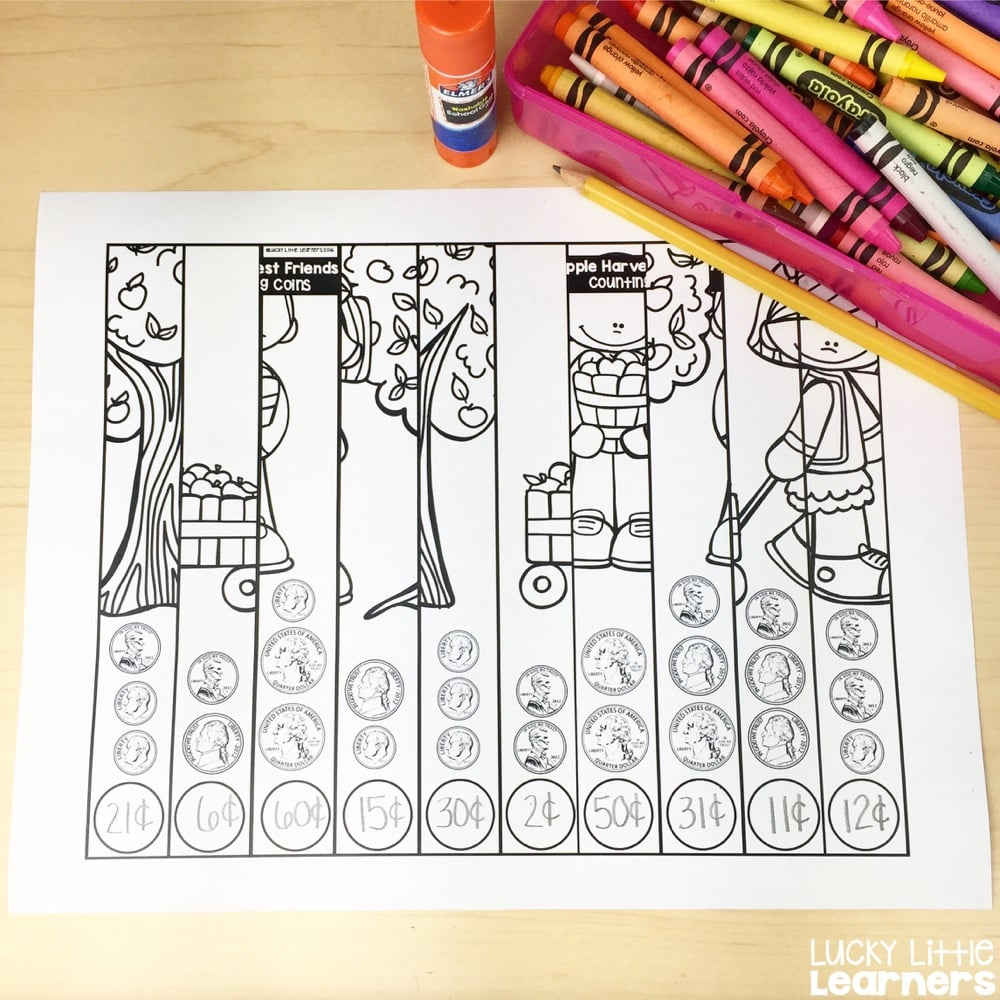## Student Accountability

Teachers who prefer to have a more structured accountability piece with the math puzzles can choose to print the recording sheets.  The student would write the name of the puzzle, which is found in the top right hand corner of the puzzle.  Then the student writes his/her answers in the bubbles on the recording sheet.

## Self Correcting Options

One of the many benefits of using these math puzzles is that they are self correcting and completely independent.  This provides the teacher with the opportunity to help other students or teach a small group.

Once the student has arranged the pieces in order from least to greatest, the student will be able to determine if the puzzle makes sense and looks right.  If not, the student would remove the piece(s) that look out of place and attempt to solve it again.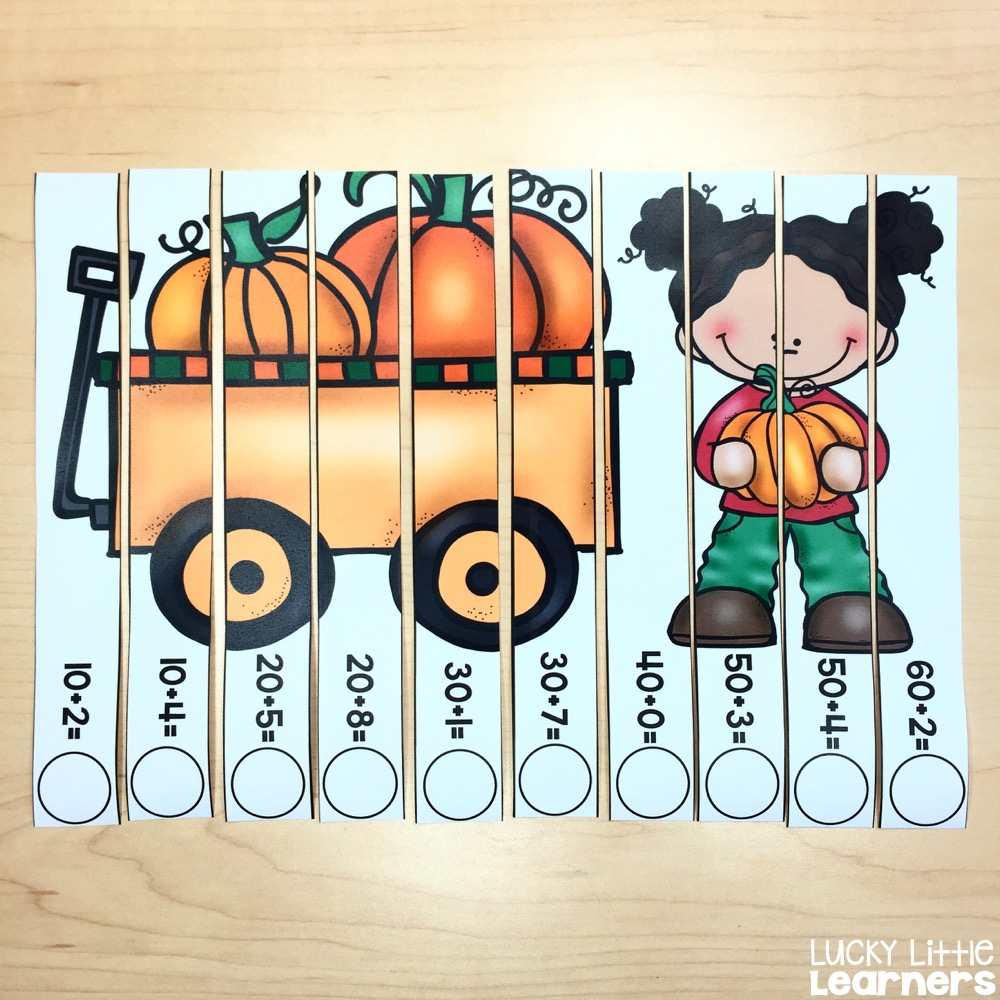## Math Puzzles Skills

### September Skills

The math skills covered in these puzzles are: addition to 20, subtraction within 20, counting coins, number rounding to 10, number rounding to 100, addition without regrouping, addition with regrouping, domino addition, multiplication, 10 more/10 less, 1 more/1 less, number bonds, number words 0-9, number words 10-19

### October Skills

The math skills covered in these puzzles are: tens & ones, addition, base ten blocks, expanded form, money(penny, nickel, dime, and quarter), subtraction, missing addends, number patterns, number sequencing, and least to greatest.

### November Skills

The math skills covered in these puzzles are: expanded form (2-digit, 3-digit, and 4-digit), basic addition facts, basic multiplication facts, odd and even (1-digit and 2-digit), value of digits (2-digit and 3-digit), missing addends (1-digit and 2-digit), number comparisons (2-digit and 3-digit), 2-digit addition (with and without regrouping), adding three numbers (1-digit and 2-digit), counting money (penny, nickel, dime, and quarter), base ten blocks (ones, tens, and hundreds), make a ten/missing addends using a ten frame, least to greatest

### December Skills

The math skills covered in these puzzles are: addition, subtraction, multiplication, time to the hour, place value (value of the underlined digit), plus and minus 1 & 10 boxes, counting coins (penny, nickel, dime, & quarter), missing addends with doubles, using doubles to subtract, fact families, skip counting (by 2’s, 3’s, 5’s, & 10’s), expanded form (2-digit and 3-digit), number bonds, 2-digit addition, 2-digit subtraction, and least to greatest

### January Skills

The math skills covered in these puzzles are: 2-Digit Subtraction (with and without regrouping), Part Part Whole, Time to the Half Hour, Fractions, Multiplication, 2-Digit Addition (with and without regrouping), Counting Coins, Doubles Facts-Addition, Subtraction, Skip Counting by 5’s, Number Bonds, 1 more/1 less, 10 more/10 less, and least to greatest

### February Skills

The math skills covered in these puzzles are: Addition, Subtraction, Multiplication, 1 More/1 Less and 10 More/10 Less Number Boxes, Counting Coins (penny, nickel, dime, quarter, and half dollar), Place Value (value of underlined digit- 2- and 3-digit numbers), Fact Families, Number Bonds, 2-Digit Addition (with and without regrouping), 2-Digit Subtraction (with and without regrouping), Part Part Whole (missing part), Telling Time to the Nearest 5 Minutes, Rounding (to nearest 10 and 100), Least to Greatest

### March Skills

The math skills covered in these puzzles are: Rounding Numbers (nearest 10 & 100), 2-Digit Addition (with & without regrouping), Addition, Subtraction, Multiplication, 1 More/1 Less, 10 More/10 Less, Place Value, Adding Three Numbers, Counting Coins (penny, nickel, dime, quarter, & half dollar), Place Value Number Boxes, Missing Addends

### April Skills

The math skills covered in these puzzles are: Addition, Subtraction, Multiplication, 2-Digit Addition (with regrouping), 2-Digit Addition (without regrouping), Number Bonds, Telling Time (nearest 5 minutes), Adding Three Numbers (1- and 2-digit), Expanded form (2- and 3-digit), Fact Families, Missing Addends, Place Value Boxes, Counting Coins, 2-Digit Subtraction (with regrouping), 2-Digit Subtraction (without regrouping), Part Part Whole and Least to Greatest

### May Skills

The math skills covered in these puzzles are: 3-Digit Addition, 3-Digit Subtraction, Adding 3 Numbers (2-digit numbers), Digit Values (2-digit and 3-digit numbers), Counting Coins (penny, nickel, dime, quarter, & half dollar), Part Part Whole, Multiplication, Fact Families, Telling Time (telling time to the nearest 5 minutes), Fractions (halves, thirds, fourths, fifths, sixths, sevenths, eighths, ninths, and tenths), Base Ten Blocks (ones, tens, hundreds), Even & Odd (2-digit and 3-digit numbers), Place Value (2-digit and 3-digit numbers), and Least to Greatest

## Math Puzzles Storage

Math puzzles can be stored in a variety of ways.  There’s not a right or wrong way to store these.  Just pick the storage method that works for your classroom.  Some of teachers’ favorite storage options include Sterilite drawers, binder rings, plastic baggies, and binder clips.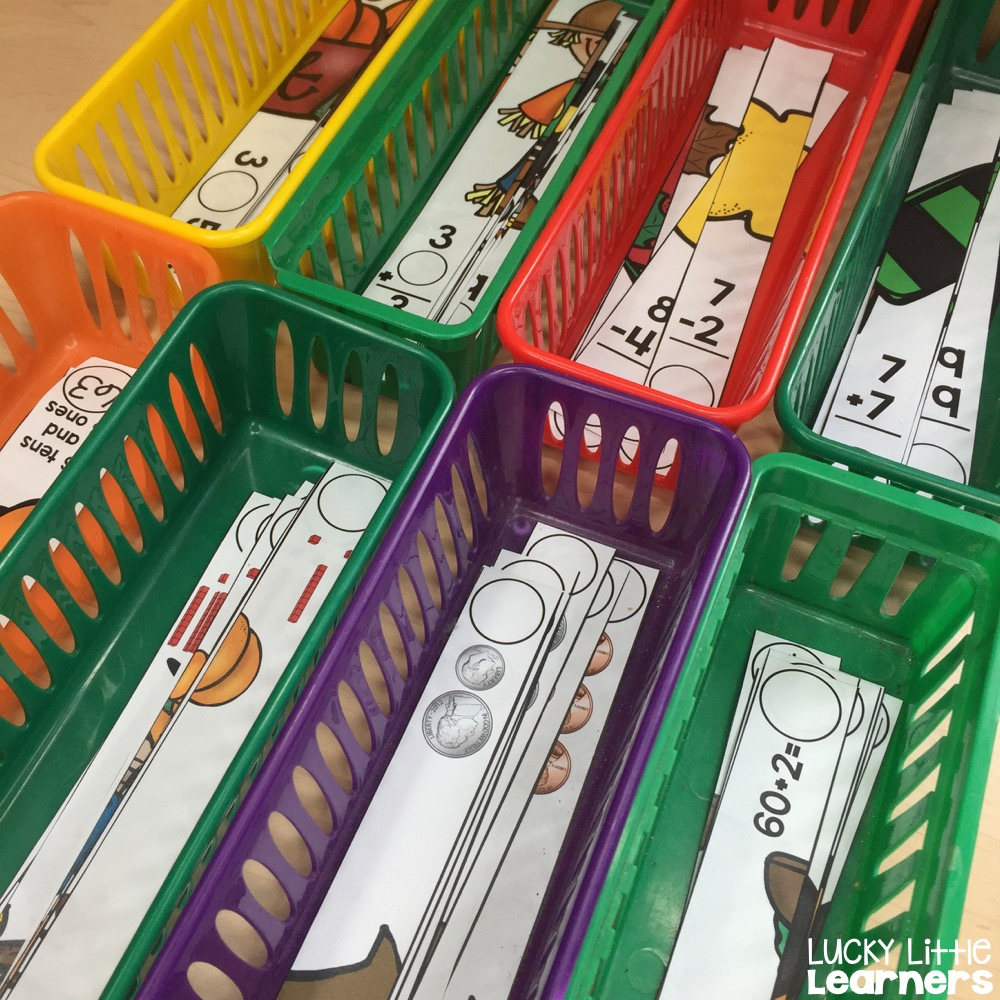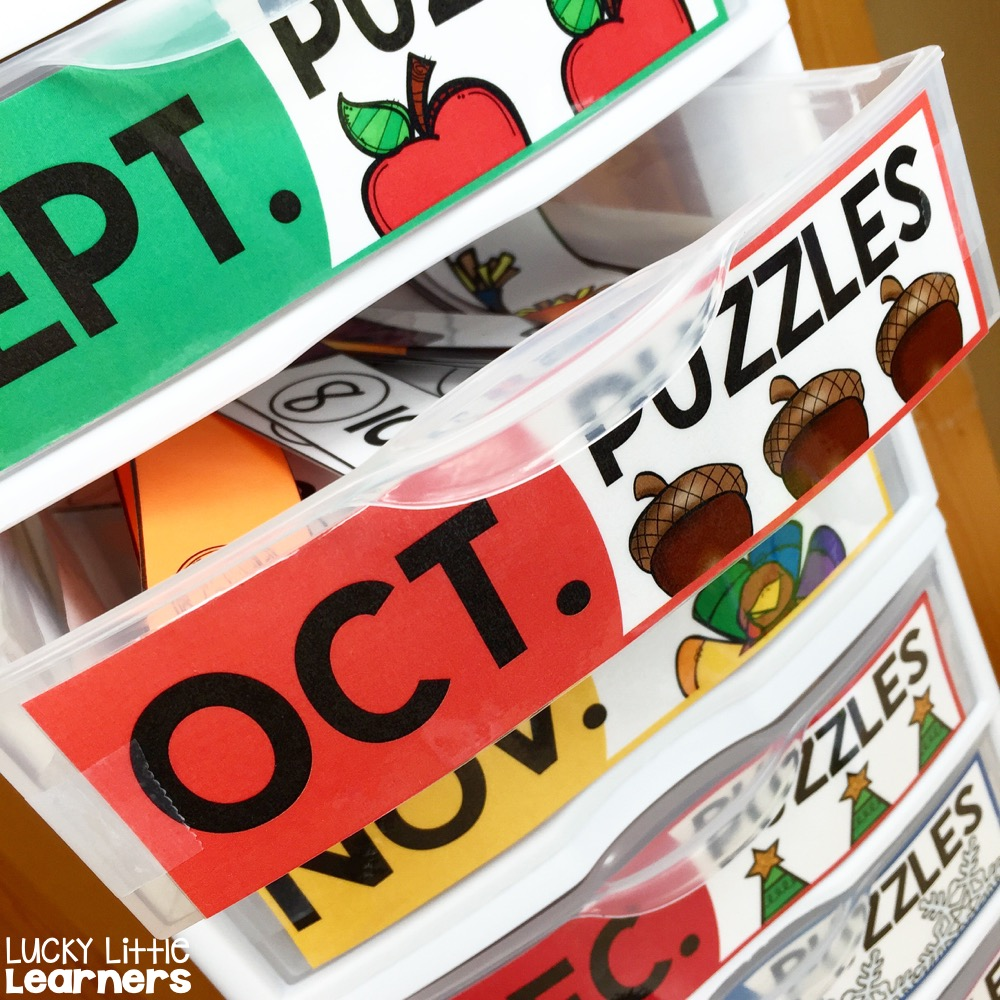## Try them out

Math puzzles are available in monthly sets or as a full year bundle.  A free set of math puzzles is also available to try out in your classroom. Click here to download the math puzzles freebie!

Click to Purchase the Monthly or Bundled Puzzles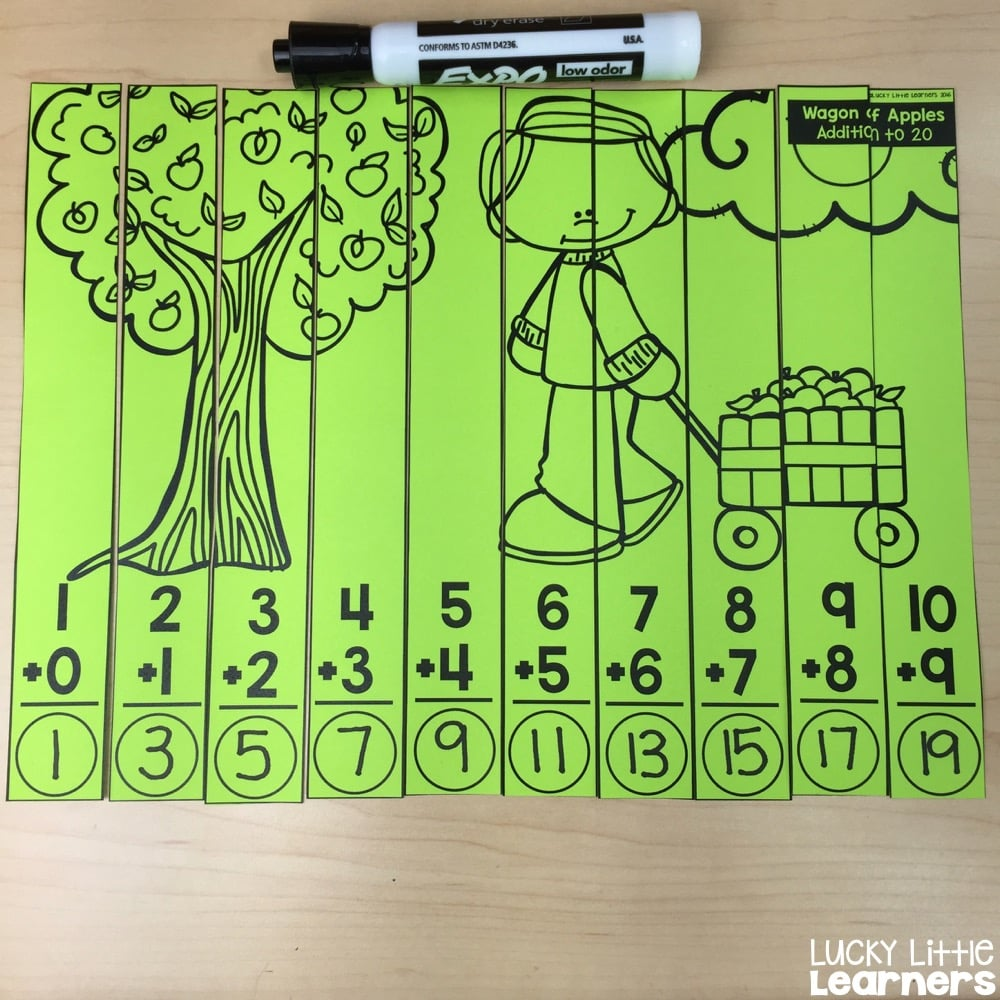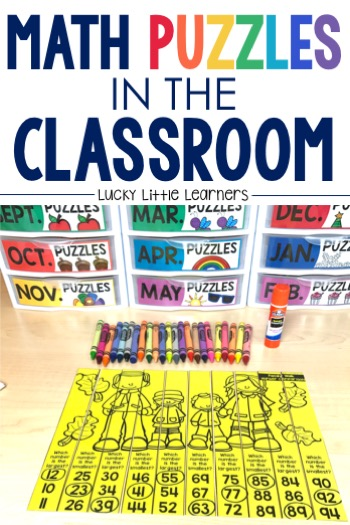#### Welcome, I’m Angie!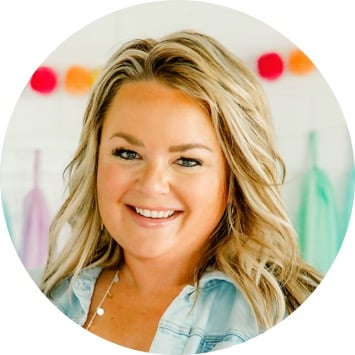Hello there! I’m Angie Olson- a teacher, curriculum developer, educational blogger and owner of Lucky Little Learners.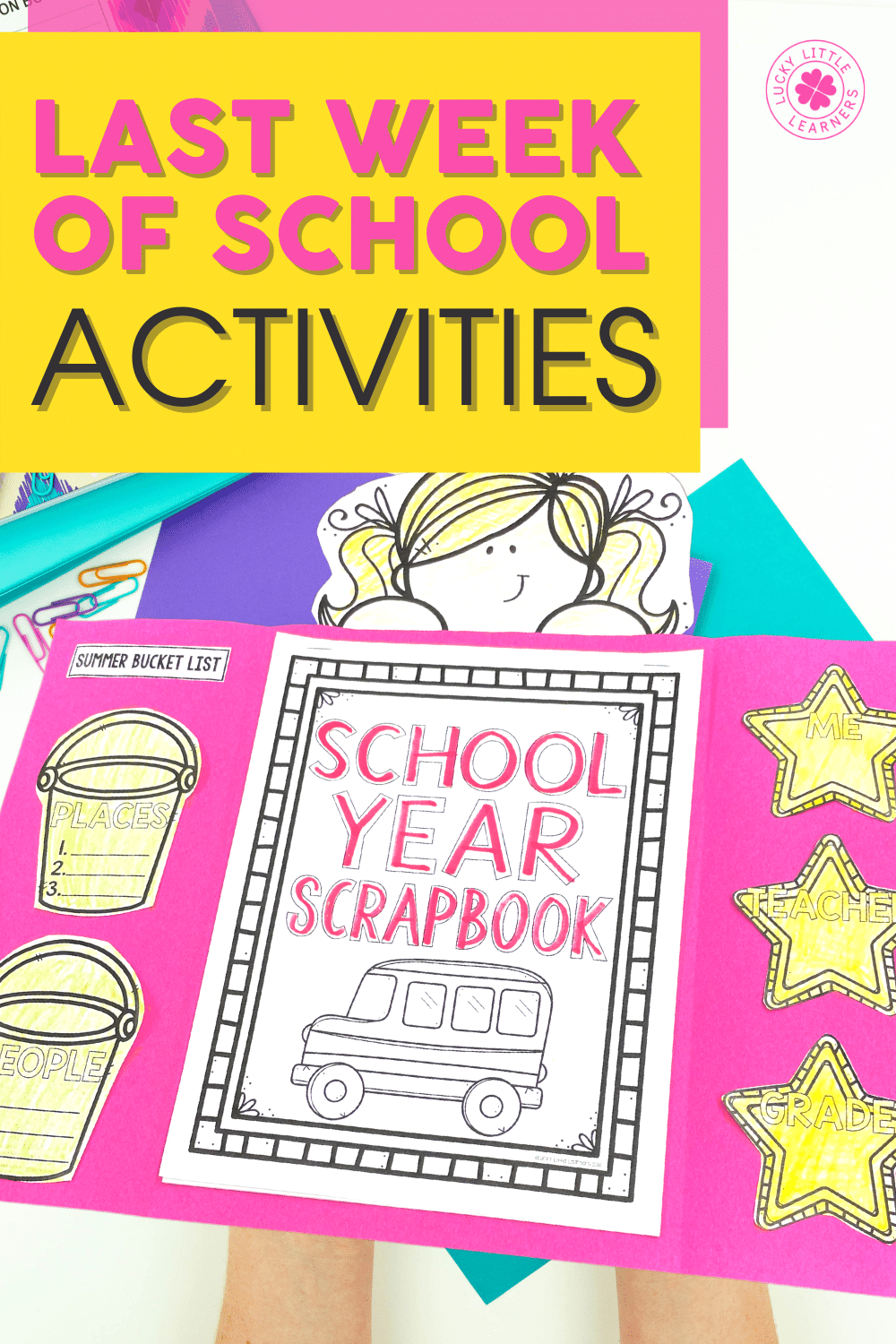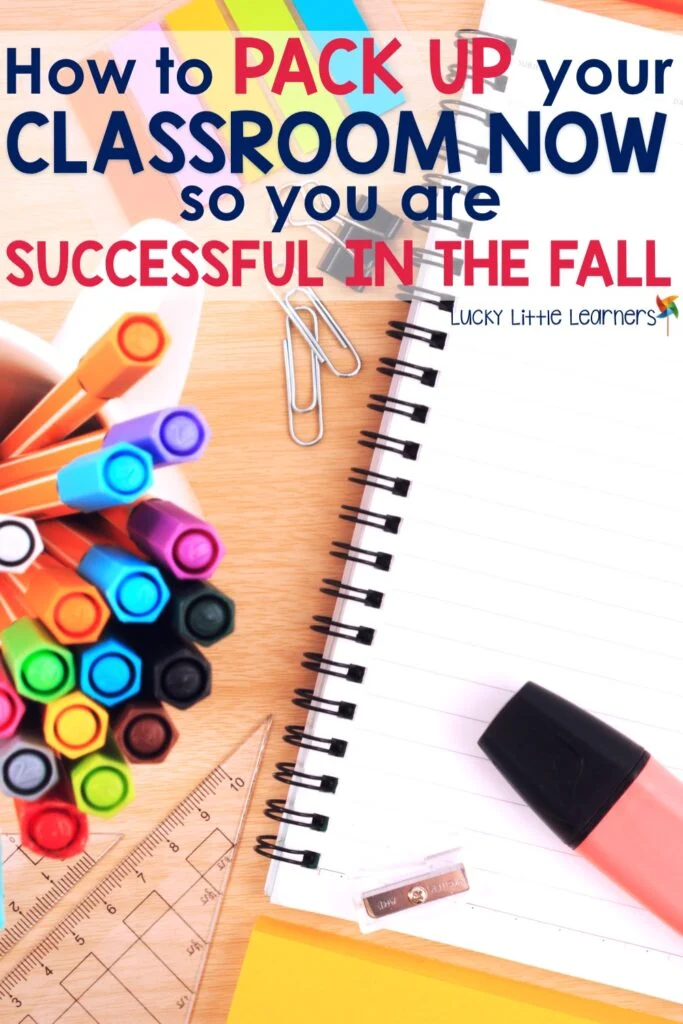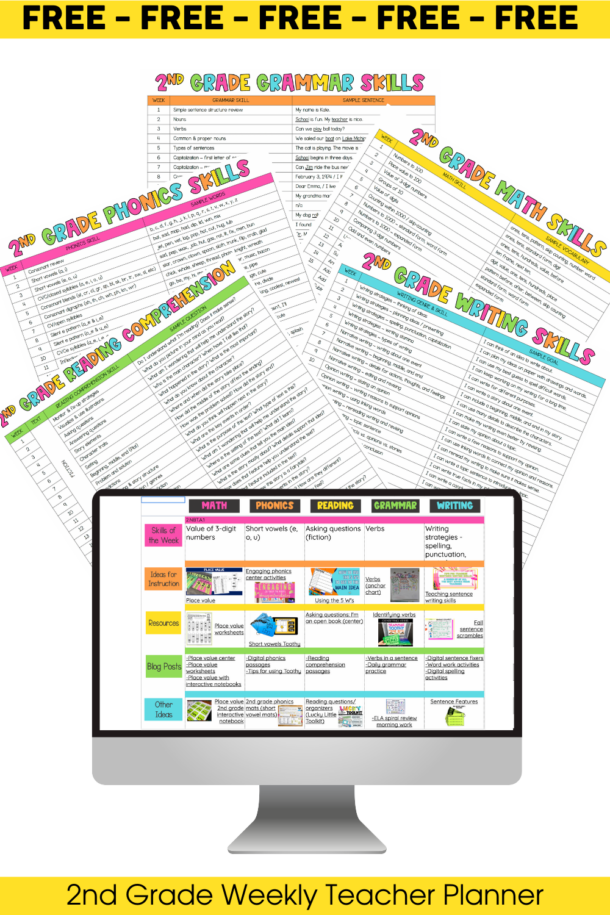## Planning is a breeze with this ⭐FREE⭐ 2nd grade scope & sequence

But that's not all! This FREEBIE also has a YEAR of free lesson plans!